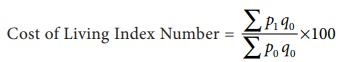Home | | Business Maths 12th Std | Construction of Cost of Living Index Number

# Construction of Cost of Living Index Number

Cost of Living Index Number is constructed to study the effect of changes in the price of goods and services of consumers for a current period as compared with base period.

Construction of Cost of Living Index Number

Cost of Living Index Number is constructed to study the effect of changes in the price of goods and services of consumers for a current period as compared with base period. The change in the cost of living index number between any two periods means the change in income which will be necessary to maintain the same standard of living in both the periods. Therefore the cost of living index number measures the average increase in the cost to maintain the same standard of life. Further, the consumption habits of people differ widely from class to class (rich, poor, middle class) and even with the region. The changes in the price level affect the different classes of people, consequently the general price index numbers fail to reflect the effect of changes in their cost of living of different classes of people. Therefore, cost of living index number measures the general price movement of the commodities consumed by different classes of people.

## Uses of Cost of Living Index Number

(i) It indicates whether the real wages of workers are rising or falling for a given time.

(ii) It is used by the administrators for regulating dearness allowance or grant of bonus to the workers.

## Methods of constructing Cost of Living Index Number

The cost of living index number can be constructed by the following methods,

(i) Aggregate Expenditure Method (or) Weighted Aggregative Method.

(ii) Family Budget Method.

## 1. Aggregate Expenditure Method

This is the most common method used to calculate cost of living index number. In this method, weights are assigned to various commodities consumed by a group in the base year. In this method the quantity of the base year is used as weight.

The formula is given by,Note

The formula for Aggregate Expenditure Method to calculate Cost of Living Index Number is same as formula of Laspeyre’s Method.

## 2. Family Budget Method

In this method, the weights are calculated by multiplying prices and quantity of the base year. (i.e.)V = p0 q0 . The formula is given by,Note

This method is same as the weighted average of price relative method.

When to Use ?

When the Price and Quantity are given, Aggregate Expenditure Method is used

When the Price and Weight are given, Family Budget Method is used.

Example 9.15

Calculate the cost of living index number for the following data.Solution:Example 9.16

Calculate the cost of living index number for the year 2015 with respect to base year 2010 of the following data.Solution:

Here the base year quantities are given, therefore we can apply Aggregate Expenditure Method.Hence, the Cost of Living Index Number for a particular class of people for the year 2015 is increased by 12.61 % as compared to the year 2010.

Example 9.17

Calculate the cost of living index number by consumer price index number for the year 2016 with respect to base year 2011 of the following data.Solution:

Here the base year quantities are given, therefore we can apply Aggregate Expenditure Method.Hence, the Cost of Living Index Number for a particular class of people for the year 2016 is increased by 59.0679 % as compared to the year 2011.

Example 9.18

Construct the cost of living index number for 2011 on the basis of 2007 from the given data using family budget method.Solution:Hence, the Cost of Living Index Number for a particular class of people for the year 2011 is increased by 25.25 % as compared to the year 2007.

Tags : Uses, Methods, Solved Example Problems | Applied Statistics , 12th Business Maths and Statistics : Chapter 9 : Applied Statistics
Study Material, Lecturing Notes, Assignment, Reference, Wiki description explanation, brief detail
12th Business Maths and Statistics : Chapter 9 : Applied Statistics : Construction of Cost of Living Index Number | Uses, Methods, Solved Example Problems | Applied Statistics